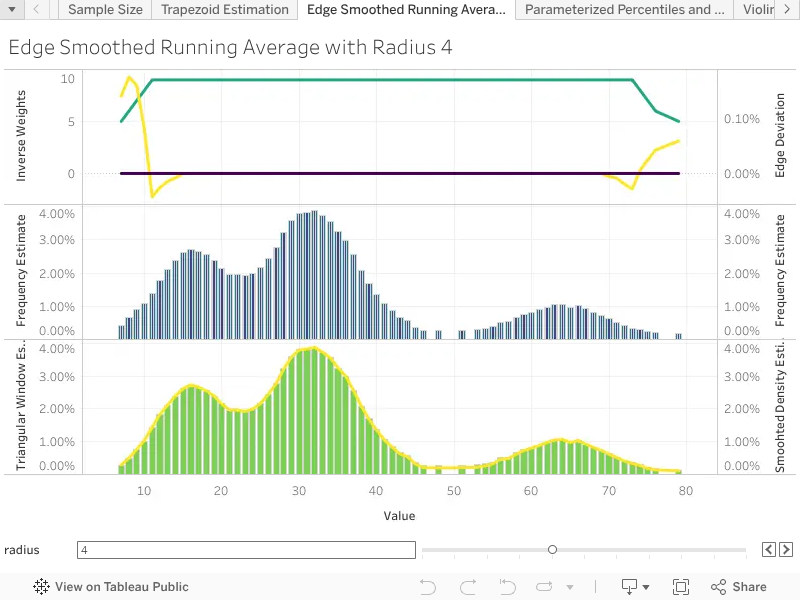# Moving Averages with Edge Smoothing by Triangular Windows

Version 10

Description:

In signal processing moving averages are an elementary technique to smooth sequential data. While Tableau's WINDOW_AVG is the simplest implementation of moving averages, being WINDOW_SUM divided by WINDOW_COUNT, it results in a smoothed data set that is inconsistent with respect to integration. For example, if the source aggregate field is a relative frequency that integrates to one over whole the table, then the WINDOW_AVG will only integrate to one for windows with zero radius, coinciding exactly with each single point of the aggregate field. This occurs because points near the edge of the table, within the twice the WINDOWS_AVG radius to the edge of the table, are over weighted compared to points sufficiently far from the edges. Specifically, points in the middle have weights that integrate to a constant with respect to the radius, whereas points near the edges have weights that integrate to a function that grows in logarithmic order with respect to the radius. To see this, begin by assuming the bare relative frequencies in each row sum to 1.

Next consider the sum over all the windows of a window average and inspect the terms for each row.

The previous equation highlights two compounding problems with the naive implementation of WINDOW_AVG. First, the size of the window decreases on the edges, and so the relative weights of the points near the edges increases. Second, the points on the edges fall in fewer windows then the points in the middle, but not enough to compensate for the growing weights. The simplest method to restore consistency with respect to integration is to weight each point inversely proportional to the number of windows in which the point belongs. This can be implemented by using a triangular window function at the edges of the table. For points in the middle this re-weighting results in no change in the running average, only points near the edges have their weight decreased appropriately. The triangular window function has a pleasantly concise implementation in Tableau, by recognising that FIRST and LAST functions measure the distances to the edges of the table. After the triangular window function is specified, all that remains is to replace the WINDOW_AVG with a WINDOW_SUM over the points of the aggregate field, each re-weighted by the inverse of the triangular window function.

Example Calculation:

// Weight each point in the window average inversely proportional

// to the number of windows in which the point resides .

[SMOOTH] := WINDOW_SUM(

)

In the case of record count frequencies, we can simplify the calculation by exploiting the linearity of the WINDOW_SUM to move the total outside of the sum.

// Weight each point in the window average inversely proportional

// to the number of windows in which the point resides .

[SMOOTH] := WINDOW_SUM(

) / TOTAL(SUM([Number of Records]))

Finally in the case of density estimation, implicit when the aggregates are plotted using a line type mark, we divide by the total length of the interval in which each point is integrated.

// Weight each point in the window average inversely proportional to

// the total length of the axis in which the point is integrated

[SMOOTH] := 2 * WINDOW_SUM(

SUM([Number of Records]) / (

(

IFNULL(LOOKUP(ATTR([ORDER]), 1 + [RADIUS]), LOOKUP(ATTR([ORDER]), LAST()))

) - (

IFNULL(LOOKUP(ATTR([ORDER]), -(1 + [RADIUS])), LOOKUP(ATTR([ORDER])), FIRST())

)

),

) / TOTAL(SUM([Number of Records]))

This final running average is mathematically consistent with respect to integration on along the axis of the dimension, in that the trapezoids will integrate to 1.

Inputs and Setup:

This table calculation is computed on any [AGGREGATE] field or expression, or any field that results in a valid ATTR output with respect to the field that the table function addresses.  The [RADIUS] can be any non-negative integer, but is best specified as a non-negative integer parameter.

A field that specifies the how the table function will be addressed is required. Additional partitions can be added as desired.

Tableau calculations do not support recursion so more sophisticated non-linear kernels will require a call to an external R instance.

Related Functions:

WINDOW_SUM, MIN, FIRST, LAST, LOOKUP